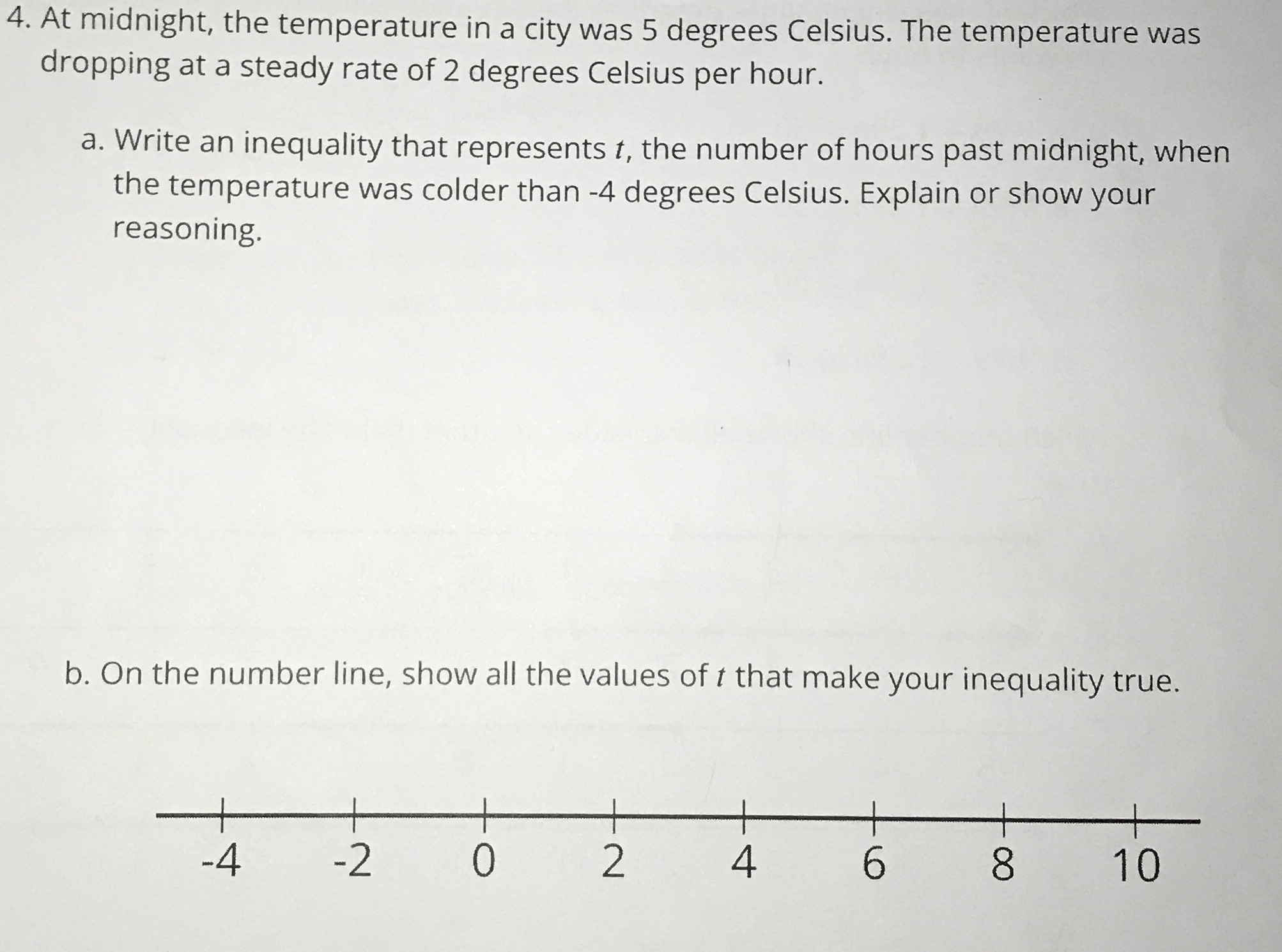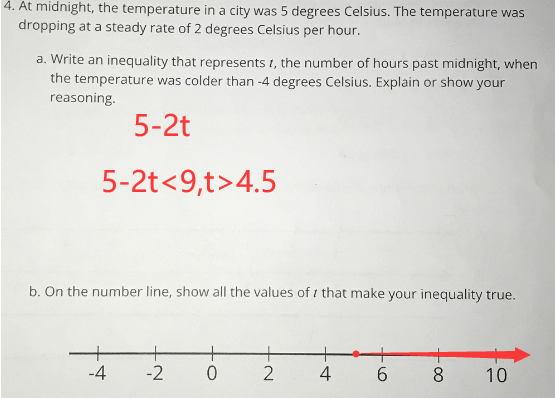### Still have math questions?

Algebra
QuestionAt midnight, the temperature in a city was $$5$$ degrees Celsius. The temperature was dropping at a steady rate of $$2$$ degrees Celsius per hour.

a. Write an inequality that represents $$t$$ , the number of hours past midnight, when the temperature was colder than $$- 4$$ degrees Celsius. Explain or show your reasoning.

b. On the number line, show all the values of $$t$$ that make your inequality true.